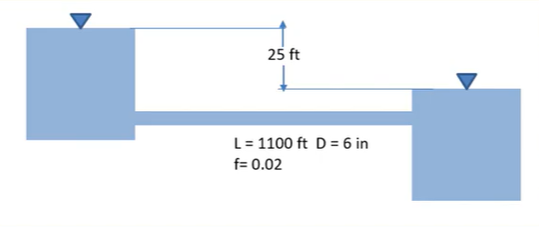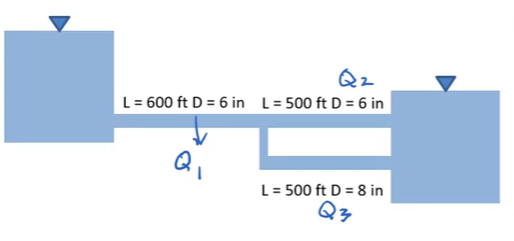#### Multipipe Systems: Example Problems

Try to solve these problems before watching the solutions in the screencasts.

##### Example Problem 1

An incompressible fluid with a density of 0.00209 slugs/ft3 flows from a tank at 0.5 psi through a 20 ft long pipe of 1 inch diameter and then through a pipe 0.5 in diameter also 20 feet long. If the friction factor for both pipes is 0.015, what is the flowrate? Assume the pipe exits into the atmosphere and neglect minor losses.

##### Example Problem 2

Calculate the flowrates in these two systems, then calculate the percentage change in flowrate between the two systems.